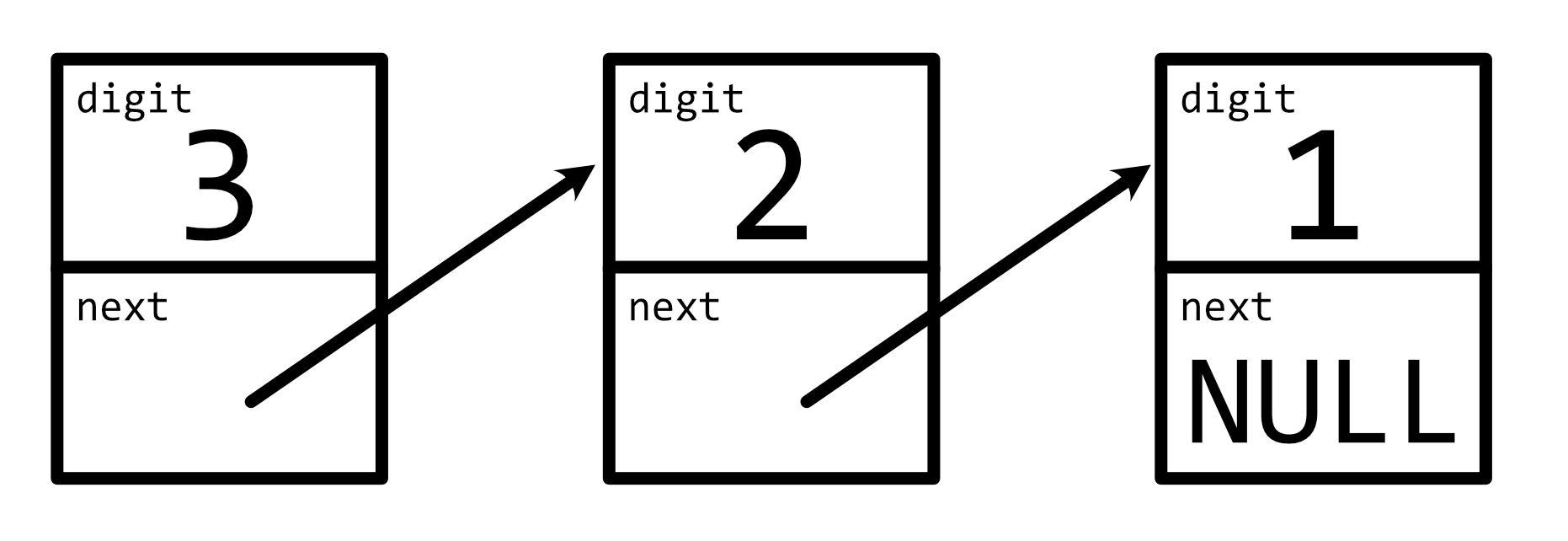# Baby Shark Dance

On November 2, 2020, Baby Shark Dance surpassed Despacito to become the most-watched YouTube video of all time, with more than 7 billion views.

Suppose that YouTube itself is implemented in C.

1. (1 point.) In no more than three sentences, what problem might YouTube run into if using an int to store each video’s number of views?

One solution to that problem is to use “arbitrary-precision integers” that can represent integers of any size. In C, for instance, we could use a linked list to implement arbitrary-precision integers by defining a new type as follows:

typedef struct node
{
int digit;
struct node *next;
}
node;


In this representation, each (decimal) digit of an integer is stored in a node in a linked list. Each node stores the digit itself (digit), 0 through 9, and a pointer to the next digit (next), which is NULL if there is no next digit. The digits themselves are stored in reverse order (i.e., from least-significant digit to most-significant digit). For example, 123 would be represented in memory as:Even though you couldn’t print an integer in this representation using printf alone, you could with a (recursive) function like:

void print(node *n)
{
if (n != NULL)
{
print(n->next);
printf("%i", n->digit);
}
}

1. (2 points.) Are there integers that can be represented using this representation that cannot be represented using an int alone? Provide an example or explain in no more than three sentences why no such example exists.

2. (2 points.) Are there integers that can be represented using an int alone that cannot be represented using this representation (assuming no changes to it)? Provide an example or explain in no more than three sentences why no such example exists.

Consider shark/integers.c, below, wherein each of n, a, and b is a pointer to the first node in a linked list:

#include <stdbool.h>
#include <stdlib.h>

typedef struct node
{
int digit;
struct node *next;
}
node;

bool divisible_by_three(node *n)
{
// TODO
}

bool equal(node *a, node *b)
{
// TODO
}

void increment(node *n)
{
// TODO
}

1. (3 points.) In shark/integers.c, complete the implementation of divisible_by_three in such a way that the function returns true if the integer represented by n is divisible by 3 or false if it is not. A number is divisible by 3 if and only if the sum of its digits is also divisible by 3. Assume that the sum will fit in an int. And assume that n will not be NULL. Do not alter the definition of node or the return types or parameters of any functions. And do not add additional functions to integers.c.

2. (3 points.) In shark/integers.c, complete the implementation of equal in such a way that the function returns true if the integers represented by a and b are equal (even if their pointers are different) or false if they are not. Assume that neither a nor b will be NULL. And assume that neither a nor b will have zeroes at the ends of their linked lists (which would otherwise be “leading zeroes”). Do not alter the definition of node or the return types or parameters of any functions. And do not add additional functions to integers.c.

3. (6 points.) In shark/integers.c, complete the implementation of increment in such a way that it adds 1 to the integer represented by n. For example, if n initially represents 50, then n should ultimately represent 51. Assume that malloc will not return NULL. Assume that n will not be NULL. Do not alter the definition of node or the return types or parameters of any functions. And do not add additional functions to integers.c.

You might find shark/testing.c of some help!